# 共振值测量¶

When sourcing ADXL345, be aware that there is a variety of different PCB board designs and different clones of them. Make sure that the board supports SPI mode (small number of boards appear to be hard-configured for I2C by pulling SDO to GND), and, if it is going to be connected to a 5V printer MCU, that it has a voltage regulator and a level shifter.

## 安装指南¶

### 接线¶

3V3 或 VCC 01 3.3v 直流（DC）电源
GND 06 地（GND）
CS（芯片选定） 24 GPIO08 (SPI0_CE0_N)
SDO 21 GPIO09 (SPI0_MISO)
SDA 19 GPIO10 (SPI0_MOSI)
SCL 23 GPIO11 (SPI0_SCLK)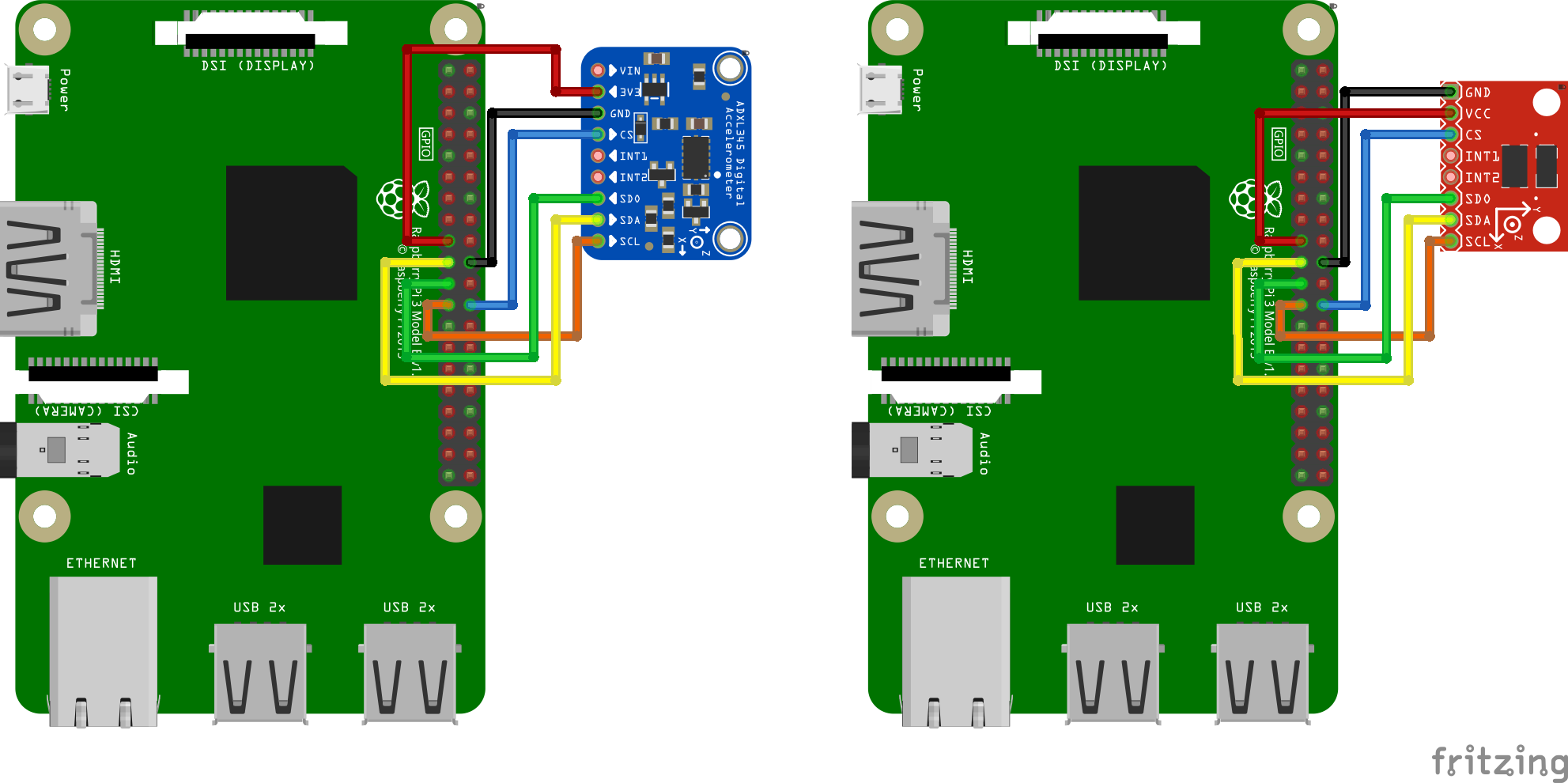### 固定加速度传感器¶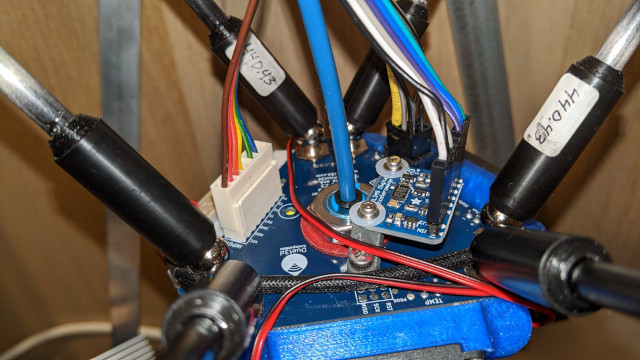### 软件设置¶

``````~/klippy-env/bin/pip install -v numpy
``````

Next, run the following commands to install the additional dependencies:

``````sudo apt update
sudo apt install python3-numpy python3-matplotlib
``````

``````[mcu rpi]
serial: /tmp/klipper_host_mcu

cs_pin: rpi:None

[resonance_tester]
probe_points:
100, 100, 20  # an example
``````

## 测量共振值¶

### 检查设置¶

• 对于只有一个加速度传感器的情况，在Octoprint，输入`ACCELEROMETER_QUERY`（遍历已连接的加速度传感器）
• 对于“滑动床”（即有多个加速度传感器），输入`ACCELEROMETER_QUERY CHIP=<chip>`，其中`<chip>`是设置文档中的加速度传感器命名，例如 `CHIP=bed`(参见：bed-slinger)。

``````Recv: // adxl345 values (x, y, z): 470.719200, 941.438400, 9728.196800
``````

### 测量共振值¶

``````TEST_RESONANCES AXIS=X
``````

``````[resonance_tester]
accel_per_hz: 50  # default is 75
probe_points: ...
``````

``````TEST_RESONANCES AXIS=Y
``````

This will generate 2 CSV files (`/tmp/resonances_x_*.csv` and `/tmp/resonances_y_*.csv`). These files can be processed with the stand-alone script on a Raspberry Pi. To do that, run the following commands:

``````~/klipper/scripts/calibrate_shaper.py /tmp/resonances_x_*.csv -o /tmp/shaper_calibrate_x.png
~/klipper/scripts/calibrate_shaper.py /tmp/resonances_y_*.csv -o /tmp/shaper_calibrate_y.png
``````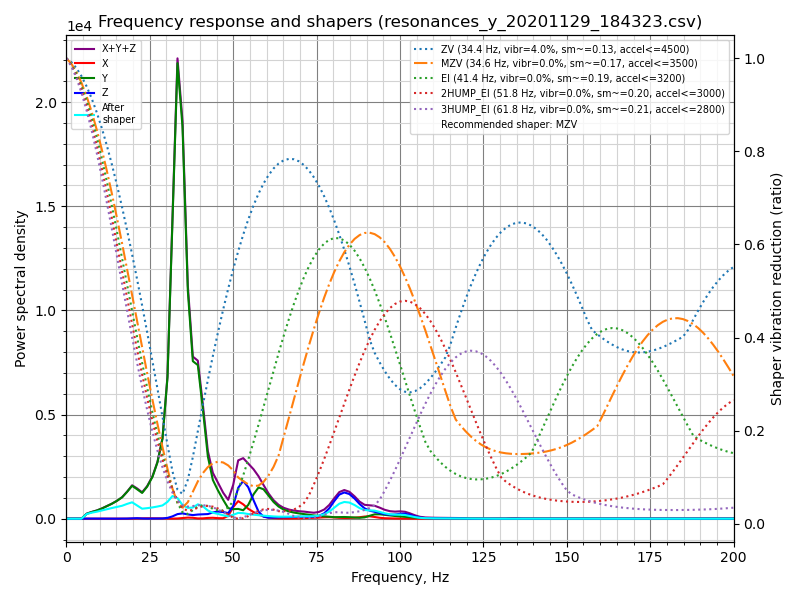``````Fitted shaper 'zv' frequency = 34.4 Hz (vibrations = 4.0%, smoothing ~= 0.132)
To avoid too much smoothing with 'zv', suggested max_accel <= 4500 mm/sec^2
Fitted shaper 'mzv' frequency = 34.6 Hz (vibrations = 0.0%, smoothing ~= 0.170)
To avoid too much smoothing with 'mzv', suggested max_accel <= 3500 mm/sec^2
Fitted shaper 'ei' frequency = 41.4 Hz (vibrations = 0.0%, smoothing ~= 0.188)
To avoid too much smoothing with 'ei', suggested max_accel <= 3200 mm/sec^2
Fitted shaper '2hump_ei' frequency = 51.8 Hz (vibrations = 0.0%, smoothing ~= 0.201)
To avoid too much smoothing with '2hump_ei', suggested max_accel <= 3000 mm/sec^2
Fitted shaper '3hump_ei' frequency = 61.8 Hz (vibrations = 0.0%, smoothing ~= 0.215)
To avoid too much smoothing with '3hump_ei', suggested max_accel <= 2800 mm/sec^2
Recommended shaper is mzv @ 34.6 Hz
``````

``````[input_shaper]
shaper_freq_x: ...
shaper_type_x: ...
shaper_freq_y: 34.6
shaper_type_y: mzv

[printer]
max_accel: 3000  # should not exceed the estimated max_accel for X and Y axes
``````

### 平行于喷嘴移动打印床的打印机¶

However, you can also connect two accelerometers simultaneously, though they must be connected to different boards (say, to an RPi and printer MCU board), or to two different physical SPI interfaces on the same board (rarely available). Then they can be configured in the following manner:

``````[adxl345 hotend]
# Assuming `hotend` chip is connected to an RPi
cs_pin: rpi:None

# Assuming `bed` chip is connected to a printer MCU board
cs_pin: ...  # Printer board SPI chip select (CS) pin

[resonance_tester]
# Assuming the typical setup of the bed slinger printer
probe_points: ...
``````

Then the commands `TEST_RESONANCES AXIS=X` and `TEST_RESONANCES AXIS=Y` will use the correct accelerometer for each axis.

### Max smoothing¶

Keep in mind that the input shaper can create some smoothing in parts. Automatic tuning of the input shaper performed by `calibrate_shaper.py` script or `SHAPER_CALIBRATE` command tries not to exacerbate the smoothing, but at the same time they try to minimize the resulting vibrations. Sometimes they can make a sub-optimal choice of the shaper frequency, or maybe you simply prefer to have less smoothing in parts at the expense of a larger remaining vibrations. In these cases, you can request to limit the maximum smoothing from the input shaper.

Let's consider the following results from the automatic tuning: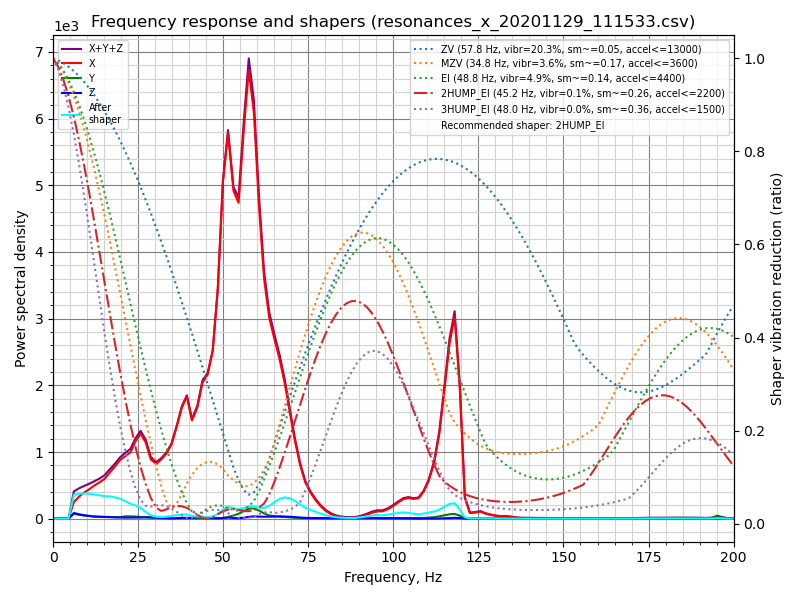``````Fitted shaper 'zv' frequency = 57.8 Hz (vibrations = 20.3%, smoothing ~= 0.053)
To avoid too much smoothing with 'zv', suggested max_accel <= 13000 mm/sec^2
Fitted shaper 'mzv' frequency = 34.8 Hz (vibrations = 3.6%, smoothing ~= 0.168)
To avoid too much smoothing with 'mzv', suggested max_accel <= 3600 mm/sec^2
Fitted shaper 'ei' frequency = 48.8 Hz (vibrations = 4.9%, smoothing ~= 0.135)
To avoid too much smoothing with 'ei', suggested max_accel <= 4400 mm/sec^2
Fitted shaper '2hump_ei' frequency = 45.2 Hz (vibrations = 0.1%, smoothing ~= 0.264)
To avoid too much smoothing with '2hump_ei', suggested max_accel <= 2200 mm/sec^2
Fitted shaper '3hump_ei' frequency = 48.0 Hz (vibrations = 0.0%, smoothing ~= 0.356)
To avoid too much smoothing with '3hump_ei', suggested max_accel <= 1500 mm/sec^2
Recommended shaper is 2hump_ei @ 45.2 Hz
``````

Note that the reported `smoothing` values are some abstract projected values. These values can be used to compare different configurations: the higher the value, the more smoothing a shaper will create. However, these smoothing scores do not represent any real measure of smoothing, because the actual smoothing depends on `max_accel` and `square_corner_velocity` parameters. Therefore, you should print some test prints to see how much smoothing exactly a chosen configuration creates.

In the example above the suggested shaper parameters are not bad, but what if you want to get less smoothing on the X axis? You can try to limit the maximum shaper smoothing using the following command:

``````~/klipper/scripts/calibrate_shaper.py /tmp/resonances_x_*.csv -o /tmp/shaper_calibrate_x.png --max_smoothing=0.2
``````

which limits the smoothing to 0.2 score. Now you can get the following result: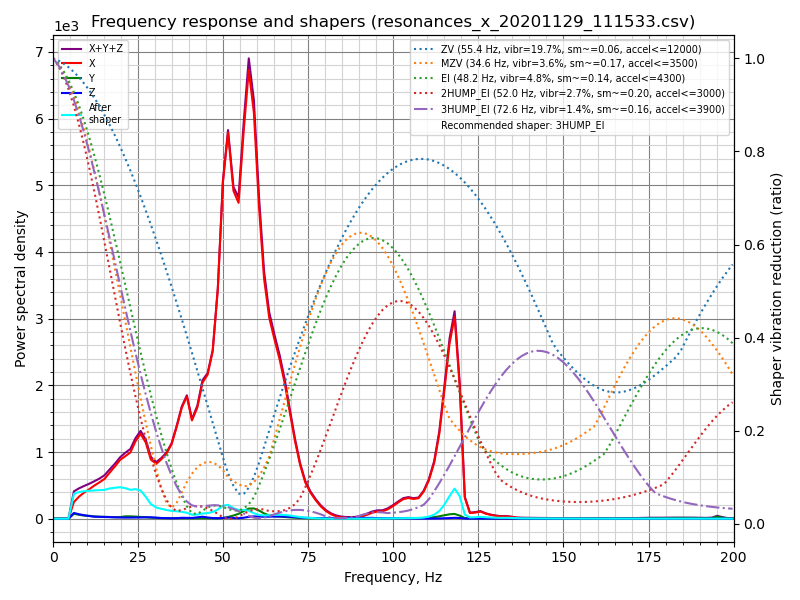``````Fitted shaper 'zv' frequency = 55.4 Hz (vibrations = 19.7%, smoothing ~= 0.057)
To avoid too much smoothing with 'zv', suggested max_accel <= 12000 mm/sec^2
Fitted shaper 'mzv' frequency = 34.6 Hz (vibrations = 3.6%, smoothing ~= 0.170)
To avoid too much smoothing with 'mzv', suggested max_accel <= 3500 mm/sec^2
Fitted shaper 'ei' frequency = 48.2 Hz (vibrations = 4.8%, smoothing ~= 0.139)
To avoid too much smoothing with 'ei', suggested max_accel <= 4300 mm/sec^2
Fitted shaper '2hump_ei' frequency = 52.0 Hz (vibrations = 2.7%, smoothing ~= 0.200)
To avoid too much smoothing with '2hump_ei', suggested max_accel <= 3000 mm/sec^2
Fitted shaper '3hump_ei' frequency = 72.6 Hz (vibrations = 1.4%, smoothing ~= 0.155)
To avoid too much smoothing with '3hump_ei', suggested max_accel <= 3900 mm/sec^2
Recommended shaper is 3hump_ei @ 72.6 Hz
``````

If you compare to the previously suggested parameters, the vibrations are a bit larger, but the smoothing is significantly smaller than previously, allowing larger maximum acceleration.

When deciding which `max_smoothing` parameter to choose, you can use a trial-and-error approach. Try a few different values and see which results you get. Note that the actual smoothing produced by the input shaper depends, primarily, on the lowest resonance frequency of the printer: the higher the frequency of the lowest resonance - the smaller the smoothing. Therefore, if you request the script to find a configuration of the input shaper with the unrealistically small smoothing, it will be at the expense of increased ringing at the lowest resonance frequencies (which are, typically, also more prominently visible in prints). So, always double-check the projected remaining vibrations reported by the script and make sure they are not too high.

Note that if you chose a good `max_smoothing` value for both of your axes, you can store it in the `printer.cfg` as

``````[resonance_tester]
accel_chip: ...
probe_points: ...
max_smoothing: 0.25  # an example
``````

Then, if you rerun the input shaper auto-tuning using `SHAPER_CALIBRATE` Klipper command in the future, it will use the stored `max_smoothing` value as a reference.

### Selecting max_accel¶

Since the input shaper can create some smoothing in parts, especially at high accelerations, you will still need to choose the `max_accel` value that does not create too much smoothing in the printed parts. A calibration script provides an estimate for `max_accel` parameter that should not create too much smoothing. Note that the `max_accel` as displayed by the calibration script is only a theoretical maximum at which the respective shaper is still able to work without producing too much smoothing. It is by no means a recommendation to set this acceleration for printing. The maximum acceleration your printer is able to sustain depends on its mechanical properties and the maximum torque of the used stepper motors. Therefore, it is suggested to set `max_accel` in `[printer]` section that does not exceed the estimated values for X and Y axes, likely with some conservative safety margin.

Alternatively, follow this part of the input shaper tuning guide and print the test model to choose `max_accel` parameter experimentally.

The same notice applies to the input shaper auto-calibration with `SHAPER_CALIBRATE` command: it is still necessary to choose the right `max_accel` value after the auto-calibration, and the suggested acceleration limits will not be applied automatically.

If you are doing a shaper re-calibration and the reported smoothing for the suggested shaper configuration is almost the same as what you got during the previous calibration, this step can be skipped.

### Testing custom axes¶

`TEST_RESONANCES` command supports custom axes. While this is not really useful for input shaper calibration, it can be used to study printer resonances in-depth and to check, for example, belt tension.

To check the belt tension on CoreXY printers, execute

``````TEST_RESONANCES AXIS=1,1 OUTPUT=raw_data
TEST_RESONANCES AXIS=1,-1 OUTPUT=raw_data
``````

and use `graph_accelerometer.py` to process the generated files, e.g.

``````~/klipper/scripts/graph_accelerometer.py -c /tmp/raw_data_axis*.csv -o /tmp/resonances.png
``````

``````TEST_RESONANCES AXIS=0,1 OUTPUT=raw_data
TEST_RESONANCES AXIS=-0.866025404,-0.5 OUTPUT=raw_data
TEST_RESONANCES AXIS=0.866025404,-0.5 OUTPUT=raw_data
``````

``````~/klipper/scripts/graph_accelerometer.py -c /tmp/raw_data_axis*.csv -o /tmp/resonances.png
``````

## Input Shaper auto-calibration¶

Besides manually choosing the appropriate parameters for the input shaper feature, it is also possible to run the auto-tuning for the input shaper directly from Klipper. Run the following command via Octoprint terminal:

``````SHAPER_CALIBRATE
``````

This will run the full test for both axes and generate the csv output (`/tmp/calibration_data_*.csv` by default) for the frequency response and the suggested input shapers. You will also get the suggested frequencies for each input shaper, as well as which input shaper is recommended for your setup, on Octoprint console. For example:

``````Calculating the best input shaper parameters for y axis # 正在计算y轴的最佳输入整形参数
Fitted shaper 'zv' frequency = 39.0 Hz (vibrations = 13.2%, smoothing ~= 0.105) # 拟合整形“zv”
To avoid too much smoothing with 'zv', suggested max_accel <= 5900 mm/sec^2 # 为避免使用“zv”方法产生过度平滑，建议最大加速度<=5900 mm/sec^2
Fitted shaper 'mzv' frequency = 36.8 Hz (vibrations = 1.7%, smoothing ~= 0.150) # 拟合整形“mzv”
To avoid too much smoothing with 'mzv', suggested max_accel <= 4000 mm/sec^2 # 为避免使用“mzv”方法产生过度平滑，建议最大加速度<=4000 mm/sec^2
Fitted shaper 'ei' frequency = 36.6 Hz (vibrations = 2.2%, smoothing ~= 0.240) # 拟合整形“ei”
To avoid too much smoothing with 'ei', suggested max_accel <= 2500 mm/sec^2 # 为避免使用“ei”方法产生过度平滑，建议最大加速度<=2500 mm/sec^2
Fitted shaper '2hump_ei' frequency = 48.0 Hz (vibrations = 0.0%, smoothing ~= 0.234) # 拟合整形“2hump_ei”
To avoid too much smoothing with '2hump_ei', suggested max_accel <= 2500 mm/sec^2 # 为避免使用“2hump_ei”方法产生过度平滑，建议最大加速度<=2500 mm/sec^2
Fitted shaper '3hump_ei' frequency = 59.0 Hz (vibrations = 0.0%, smoothing ~= 0.235) # 拟合整形“3hump_ei”
To avoid too much smoothing with '3hump_ei', suggested max_accel <= 2500 mm/sec^2 # 为避免使用“3hump_ei”方法产生过度平滑，建议最大加速度<=2500 mm/sec^2
Recommended shaper_type_y = mzv, shaper_freq_y = 36.8 Hz # 建议shaper_type_y = mzv, shaper_freq_y = 36.8 Hz
``````

If you agree with the suggested parameters, you can execute `SAVE_CONFIG` now to save them and restart the Klipper. Note that this will not update `max_accel` value in `[printer]` section. You should update it manually following the considerations in Selecting max_accel section.

If your printer is a bed slinger printer, you can specify which axis to test, so that you can change the accelerometer mounting point between the tests (by default the test is performed for both axes):

``````SHAPER_CALIBRATE AXIS=Y
``````

You can execute `SAVE_CONFIG` twice - after calibrating each axis.

However, if you connected two accelerometers simultaneously, you simply run `SHAPER_CALIBRATE` without specifying an axis to calibrate the input shaper for both axes in one go.

### Input Shaper re-calibration¶

`SHAPER_CALIBRATE` command can be also used to re-calibrate the input shaper in the future, especially if some changes to the printer that can affect its kinematics are made. One can either re-run the full calibration using `SHAPER_CALIBRATE` command, or restrict the auto-calibration to a single axis by supplying `AXIS=` parameter, like

``````SHAPER_CALIBRATE AXIS=X
``````

Warning! It is not advisable to run the shaper autocalibration very frequently (e.g. before every print, or every day). In order to determine resonance frequencies, autocalibration creates intensive vibrations on each of the axes. Generally, 3D printers are not designed to withstand a prolonged exposure to vibrations near the resonance frequencies. Doing so may increase wear of the printer components and reduce their lifespan. There is also an increased risk of some parts unscrewing or becoming loose. Always check that all parts of the printer (including the ones that may normally not move) are securely fixed in place after each auto-tuning.

Also, due to some noise in measurements, it is possible that the tuning results will be slightly different from one calibration run to another one. Still, it is not expected that the noise will affect the print quality too much. However, it is still advised to double-check the suggested parameters, and print some test prints before using them to confirm they are good.

## Offline processing of the accelerometer data¶

It is possible to generate the raw accelerometer data and process it offline (e.g. on a host machine), for example to find resonances. In order to do so, run the following commands via Octoprint terminal:

``````SET_INPUT_SHAPER SHAPER_FREQ_X=0 SHAPER_FREQ_Y=0
TEST_RESONANCES AXIS=X OUTPUT=raw_data
``````

ignoring any errors for `SET_INPUT_SHAPER` command. For `TEST_RESONANCES` command, specify the desired test axis. The raw data will be written into `/tmp` directory on the RPi.

The raw data can also be obtained by running the command `ACCELEROMETER_MEASURE` command twice during some normal printer activity - first to start the measurements, and then to stop them and write the output file. Refer to G-Codes for more details.

The data can be processed later by the following scripts: `scripts/graph_accelerometer.py` and `scripts/calibrate_shaper.py`. Both of them accept one or several raw csv files as the input depending on the mode. The graph_accelerometer.py script supports several modes of operation:

• plotting raw accelerometer data (use `-r` parameter), only 1 input is supported;
• plotting a frequency response (no extra parameters required), if multiple inputs are specified, the average frequency response is computed;
• comparison of the frequency response between several inputs (use `-c` parameter); you can additionally specify which accelerometer axis to consider via `-a x`, `-a y` or `-a z` parameter (if none specified, the sum of vibrations for all axes is used);
• plotting the spectrogram (use `-s` parameter), only 1 input is supported; you can additionally specify which accelerometer axis to consider via `-a x`, `-a y` or `-a z` parameter (if none specified, the sum of vibrations for all axes is used).

Note that graph_accelerometer.py script supports only the raw_data*.csv files and not resonances*.csv or calibration_data*.csv files.

For example,

``````~/klipper/scripts/graph_accelerometer.py /tmp/raw_data_x_*.csv -o /tmp/resonances_x.png -c -a z
``````

will plot the comparison of several `/tmp/raw_data_x_*.csv` files for Z axis to `/tmp/resonances_x.png` file.

The shaper_calibrate.py script accepts 1 or several inputs and can run automatic tuning of the input shaper and suggest the best parameters that work well for all provided inputs. It prints the suggested parameters to the console, and can additionally generate the chart if `-o output.png` parameter is provided, or the CSV file if `-c output.csv` parameter is specified.

Providing several inputs to shaper_calibrate.py script can be useful if running some advanced tuning of the input shapers, for example:

• Running `TEST_RESONANCES AXIS=X OUTPUT=raw_data` (and `Y` axis) for a single axis twice on a bed slinger printer with the accelerometer attached to the toolhead the first time, and the accelerometer attached to the bed the second time in order to detect axes cross-resonances and attempt to cancel them with input shapers.
• Running `TEST_RESONANCES AXIS=Y OUTPUT=raw_data` twice on a bed slinger with a glass bed and a magnetic surfaces (which is lighter) to find the input shaper parameters that work well for any print surface configuration.
• Combining the resonance data from multiple test points.
• Combining the resonance data from 2 axis (e.g. on a bed slinger printer to configure X-axis input_shaper from both X and Y axes resonances to cancel vibrations of the bed in case the nozzle 'catches' a print when moving in X axis direction).